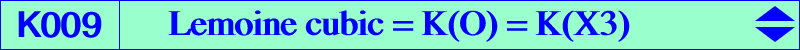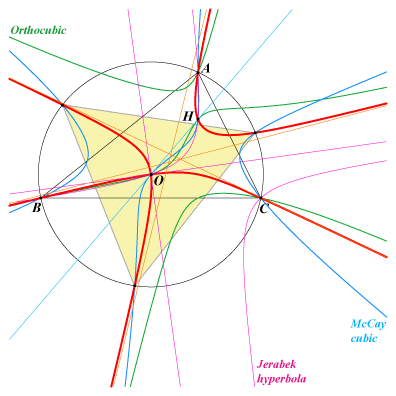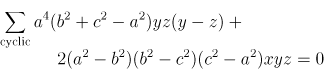X(3), X(4), X(32), X(56), X(1147), X(6177), X(6178), X(6337), X(7367), X(10570), X(11517), X(13608), X(14357), X(14376), X(14377), X(14378), X(14379), X(14381), X(14382), X(14383), X(14384), X(14385), X(14386), X(15261), X(15454), X(25044), X(34156), X(34157), X(34158), X(34159), X(34160), X(34161), X(34853), X(36944), X(38542), X(39166), X(39167), X(39168), X(39169), X(39170), X(39171), X(39172), X(39173), X(39174), X(39175), X(51346), X(51347), X(51470), X(51471), X(51472), X(51473), X(51474), X(51475), X(54233), X(54236), X(54237), X(54243) vertices of the CircumNormal triangle infinite points of the Orthocubic K006 projections of O on the altitudes A', B', C' : midpoints of ABC points Ua, Ub, Uc mentioned in the Neuberg cubic page. See also table 16 and table 18. four foci of the MacBeath inconic : X(3), X(4) and two imaginary ***In 1891, Lemoine published a note in which he very briefly studied a cubic curve defined as follows. Let M be a point in the plane of triangle ABC. Denote by Ma the intersection of the line MA with the perpendicular bisector of BC and define Mb, Mc similarly. The locus of M such that the three points Ma, Mb, Mc are collinear is the Lemoine cubic K009. More informations and generalizations are found in the FG paper "The Lemoine Cubic and Its Generalizations" where the Lemoine cubic is denoted by K(O). See also Table 64. K009 is the Psi-transform of the circumcircle and the Phi-transform of the line at infinity. See CL037. The isogonal transform of K009 is K028, the Musselman (third) cubic. These two cubics generate a pencil described below. The anticomplement of K009 is K617. The isotomic transform of K009 is psK(X18022, X18022, X69) passing through X(69), X(264), X(1502), X(3596), X(17233), X(17907), X(34208), X(40810), X(52551), X(55553). K009 is closely related to the quartic Q023. It is a member of the class CL033 (Deléham cubics). For any point Q on the line X(2)X(6), the trilinear polar of Q meets the perpendicular bisectors at Qa, Qb, Qc. ABC and QaQbQc are perspective and the perspector is a point on K009. This gives a simple way of finding a lot of reasonably simple points on the curve. K009 is also psK(X184, X2, X3) in Pseudo-Pivotal Cubics and Poristic Triangles and spK(X4, X5) in CL055. Locus properties 1. Denote by A' the intersection of the line AM with the polar of M in the circumcircle. Let La be the perpendicular at A' to BC, and Lb, Lc are defined similarly. The lines La, Lb, Lc concur (at Q) if and only if P lies on K009 (together with the line at infinity and the circumcircle). When M traverses K009, the locus of Q is a rather complicated circum-quintic passing through X(1), X(3), X(4), X(182), X(2574), X(2575), the excenters, the infinite points of the altitudes, the vertices of the CircumNormal triangle (Angel Montesdeoca, 2022-07-12, further details in Spanish here). 2. Given a triangle ABC, let L be a variable line through the circumcenter that cuts BC, CA, AB at D, E, F, respectively. The circles with diameters AD, BE, CF are coaxial and their radical axis R passes through the orthocenter. The locus of M = L ∩ R is the Lemoine cubic, K009. Let Ma be the point of intersection of AM with the perpendicular bisector of BC, and Mb, Mc, are defined cyclically. The three points Ma, Mb, Mc are on a line L', which intersects L at P. The locus of P is the Stammler strophoid K038. The envelope of L' is the Kiepert parabola (Angel Montesdeoca, 2023-10-21). Related papers The Lemoine Pencils of Cubics Another kind of Lemoine cubics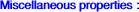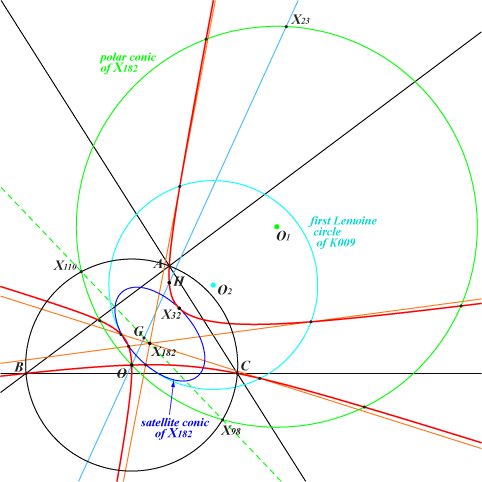X(182) is the only point whose polar conic is a circle, a point we call the Stuyvaert point of K009. This circle is the inverse in the circumcircle of the line passing through X(2), X(98), X(110) and many other ETC centers. Its center O1 is the pole of the Fermat axis in the circumcircle. O1 is also the perspector of the circum-conic passing through X(6), X(50), X(323), X(2436), X(2623). O1 is now X(14270) in ETC (2017-09-06). Recall that X(182) is the center of the Brocard circle. This circle meets K009 at X(3) twice and at four other points whose tangentials lie on the satellite conic of X(182). This conic is tangent at X(32) to K009. The parallels at X(182) to the asymptotes of K009 (and K006) meet K009 again at six (finite) points lying on a same circle, analogous to the first Lemoine circle of triangle ABC. Its center O2 is the midpoint of X(182)-O1. O2 is now X(14271) in ETC (2017-09-06).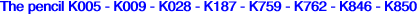Let P be the point on the Euler line such that OP = T OH (vectors) where T is a real number. Let Q be the reflection of P about the nine point center N = X(5). Any cubic of this pencil is K(T) = spK(P, N) for some T as in CL055. More specifically, see Table 54, line Q = X5 in the table. The limit case K(∞) is K187. The isogonal transform of spK(P, N) is spK(Q, N) hence the pencil is stable under isogonal conjugation. The self-isogonal cubics are K005 and K187. K(T) is a circum-cubic passing through the four foci of the MacBeath conic : two are imaginary on the line X(5)X(523), the others are X(3), X(4) each counted twice since the tangents (when defined i.e T different from 0 and 1) meet at X(74). K(T) also contains Q and the isogonal conjugate P* of P. Recall that K(T) meets the line at infinity [resp. the circumcircle] at the same points as pK(X6, P) [resp. pK(X6, Q)]. The orthic line of spK(P, N) is the Euler line except the case T = 0 corresponding to the stelloid K028. The locus of the Stuyvaert point of K(T) is the rectangular hyperbola passing through X(3), X(74), X(182), X(381), X(2574), X(2575), etc.